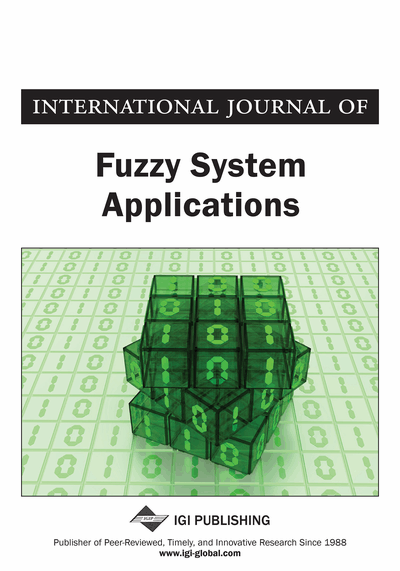# Role of Distance Metric in Goal Geometric Programming Problem (G^2 P^2) Under Imprecise Environment

Payel Ghosh (Raidighi College, West Bengal, India) and Tapan Kumar Roy (IIEST, Shibpur, Howrah, India)
DOI: 10.4018/IJFSA.2019010104
Available
\$29.50
No Current Special Offers

## Abstract

The objective of this article is to tie a knot between distance measure and fuzzy and intuitionistic fuzzy optimization through goal programming. Firstly, a distance measure for an intuitionistic fuzzy number is developed, and then it is implemented into an intuitionistic fuzzy nonlinear goal programming. Then using some conditions, the distance measure of intuitionistic fuzzy number is converted into distance measure of fuzzy number and a comparative study using a numerical example is shown for highest applicability of distance measure based intuitionistic fuzzy goal programming than distance measure based fuzzy goal programming.
Article Preview
Top

## 1. Introduction

Fuzzy means vague, imprecise. Intuitionistic fuzzy is the vagueness which comes from intuition. Real life situations which depend on human perception are better represented by intuitionistic fuzzy set (IFS). Intuitionistic fuzzy set theory was first introduced by Atanassov (1986). There are many progresses on intuitionistic fuzzy set inclusion, arithmetic operations and distance measures of intuitionistic fuzzy numbers (IFN) (Li, 2014; Zhu & Li, 2016). However, intuitionistic fuzzy optimization is a new field for researcher. Moreover, it will be better if we can join mathematical development of intuitionistic fuzzy number such as distance measure of intuitionistic fuzzy number with intuitionistic fuzzy optimization.

Most of the researchers have developed distance measures and have shown their applications taking arbitrary IFNs. They have compared their results with some existing approaches and claimed that their proposed approach is better under certain angle. Atanassov (1999), Grzegorzewski (2004), Szmidt and Kacprzyk (1997), Wang et al. (2005) have given different point of views regarding distance measure of intuitionistic fuzzy sets. Grzegorzewski (2003), Esmailzadeh et al. (2013) are few researchers who have described distance measure for IFN. Xu and Chen (2008) have described some continuous distance measure for IFS and then extend this in generalized IFS. On the other hand, there are few developments on intuitionistic fuzzy optimization. Parvathi et al. (2012, 2012) have described an algorithm for solving intuitionistic fuzzy linear programming problem (IFLPP), Nachammai et al. (2012) have brought the variation in IFLPP by using generalized IFN. Nagoorgani et al. (2012) have proposed division of triangular intuitionistic fuzzy number (TIFN) and also applied it to solve IFLPP with intuitionistic fuzzy variable. So, this is all about linear programming problem in intuitionistic fuzzy environment. However, the combination of distance measure and intuitionistic fuzzy optimization is rare. Also, few literatures are available considering nonlinearity in intuitionistic fuzzy optimization. It is a challenging issue to link between distance measure and intuitionistic fuzzy optimization. Once it is done, then its application in real world is also a difficult task.

The objective of this article is to explain the rare combination of distance measure and intuitionistic fuzzy optimization through goal programming. Goal programming tries to minimize the deviations of objective function from the targets. So, there lies a concept of distance between objective functions and their targets. We consider the case that some of the parameters of the objective functions and all of their targets are not known precisely. Then it will be an intuitionistic fuzzy goal programming problem and our aim in this paper is to incorporate distance measure into it. Moreover, a nonlinear goal programming problem is taken where coefficients are TIFN. Geometric programming is used here to tackle the nonlinearity in intuitionistic fuzzy optimization through goal programming problem. Previously intuitionistic fuzzy goal geometric programming is discussed by Ghosh, Roy (2013, 2014, 2014) with arithmetic and geometric mean operator. There, objective functions were intuitionistic fuzzy in nature. Here, distance measure is taking a great role in formulation of crisp programming from intuitionistic fuzzy goal programming problem.

## Complete Article List

Search this Journal:
Reset
Volume 12: 1 Issue (2023)
Volume 11: 4 Issues (2022)
Volume 10: 4 Issues (2021)
Volume 9: 4 Issues (2020)
Volume 8: 4 Issues (2019)
Volume 7: 4 Issues (2018)
Volume 6: 4 Issues (2017)
Volume 5: 4 Issues (2016)
Volume 4: 4 Issues (2015)
Volume 3: 4 Issues (2013)
Volume 2: 4 Issues (2012)
Volume 1: 4 Issues (2011)
View Complete Journal Contents Listing